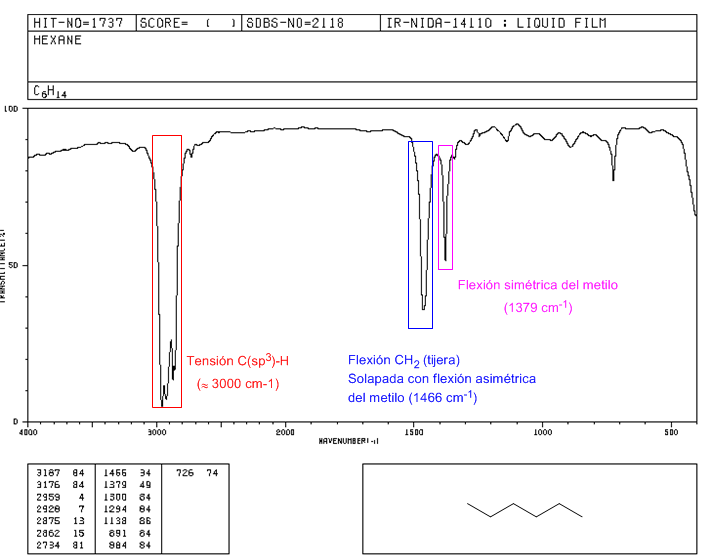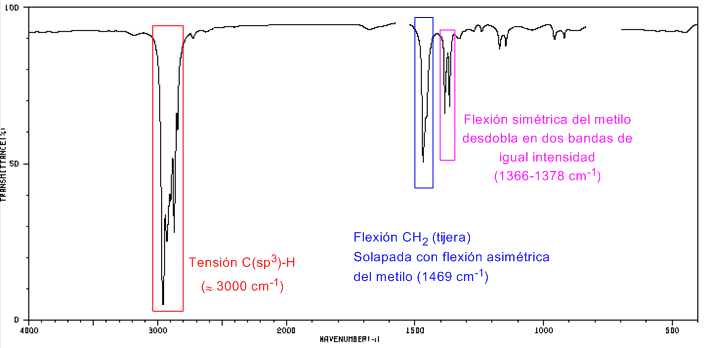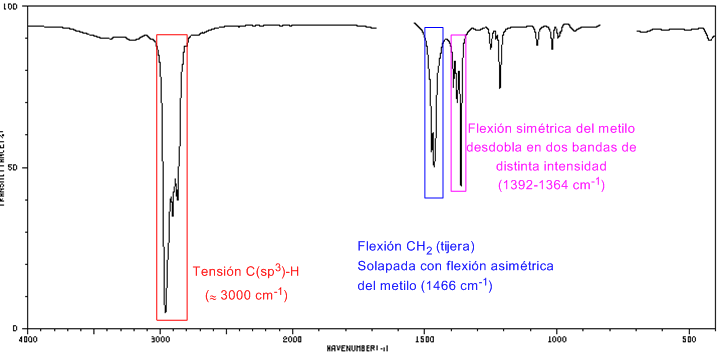• CH strain: alkanes exhibit CH strain vibrations slightly below 3000 $cm^{-1}$

• CH bending: the $CH_2$ of the chain show bending vibrations (scissors) at 1465 $cm^{-1}$, while the methyls produce a band at 1375 $cm^{-1}$ due to vibration symmetrical bending and another at 1450 $cm^{-1}$ due to asymmetrical bending vibration. All push-up bands are medium intensity.

Note that the asymmetric bending band of methyl overlaps with the scissor bending band of $CH_2$.

IR Spectrum of HexaneIR spectrum of 2-Methylpentane

The symmetric bending band of methyl splits into two bands 1380 and 1370 cm -1 of equal intensity for the isopropyl group.IR spectrum of 2,2-Dimethylbutane

The presence of the tert-butyl group produces the splitting of the symmetrical bending band into two bands at 1390 and 1370 \$cm -1 . The band at 1390 is half as strong as that at 1370.